# How to Pass Structure as a Parameter to a Function in C

## How to Pass Structure as a Parameter to a Function in C Language

In this article, we are going to discuss How to Pass Structure as a Parameter to a Function in C Language with Examples. Please read our previous article, where we discussed How to pass an Array as a Parameter to a function. If we are sending a structure as a parameter to a function, it may be called by value or call by address. So, at the end of this article, you will understand how to pass a structure to a function using call by value and call by address.

##### Passing Structure as a Parameter to a Function using Call by Value in C Language:

Let us understand how to pass a structure as a parameter to a function using the call by value mechanism in C Language. For a better understanding of this let us first create a structure as shown below. As you can see in the below image, we have a structure called Rectangle having two properties length and breadth.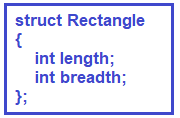Now we need to create a function called CalculateArea which should calculate the area of a rectangle. Now here, shall we pass length separate and breadth separate? No need, we can send the structure itself as a parameter and that is the benefit of using a structure. We can send the structure itself and the structure is carrying both length and breadth. For a better understanding, please have a look at the below image.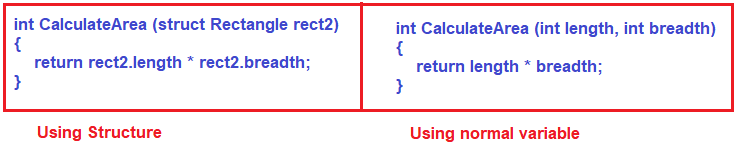Now in the main function, we need to create a variable of type structure and then we need to pass that structure as a parameter to the CalculateArea function as shown in the below image.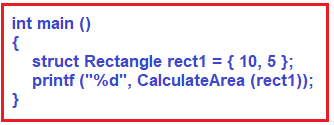##### The complete code is given below.
```#include <stdio.h>
struct Rectangle
{
int length;
};

int CalculateArea (struct Rectangle rect2)
{
}

int main ()
{
struct Rectangle rect1 = { 10, 5 };
printf ("%d", CalculateArea (rect1));
}
```
##### Code Explanation and Memory Representation:

Within the main function, first, we created a variable rect1 of type structure rectangle and it initialized it with values 10 and 5. Here 10 will be assigned to length and 20 will be assigned to breadth. Then we call the function CalculateArea by giving the structure rect1 as a parameter. In the CalculateArea function, we defined one parameter of type structure Rectangle and this parameter is called by value as we have not specified either * or &.

As we already discussed in our previous article, in passed-by value, new memory allocation will happen and the values are copied. So, here a new rectangle object rect2 will be created and the values 10 and 5 are copied to the length and breadth properties. Then the CalculateArea function calculates the area and returns that value and that value will be printed in the console window. For better understanding, please have a look at the below diagram.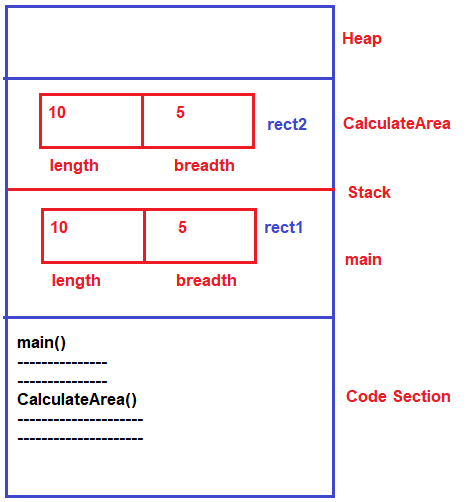Now inside the CalculateArea function, if we make changes to the rect2 object, then it will not affect the rect1 object inside the main method. This is because in the call by the value we are just passing a copy of the value. For a better understanding, please have a look at the below code.

```#include <stdio.h>
struct Rectangle
{
int length;
};

int CalculateArea (struct Rectangle rect2)
{
rect2.length = 20;
}

int main ()
{
struct Rectangle rect1 = { 10, 5 };
printf ("%d", CalculateArea (rect1));
}
```

The following image is the memory representation of the above example.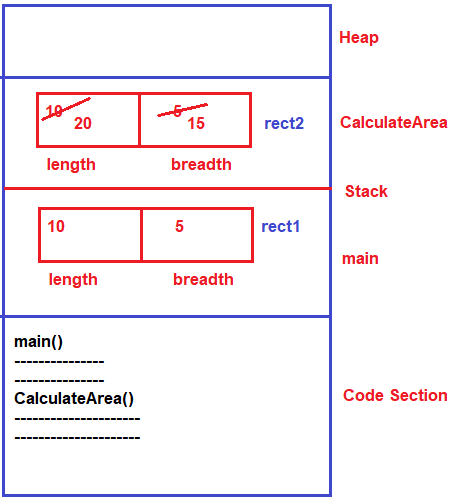##### Passing Structure as a Parameter to a Function using Call by Address in C Language

Let us understand How to Pass Structure as a Parameter to a Function using Call by Address in C Language with an example. Now our requirement is to change the length of a rectangle. We can change the length itself within the main function but we don’t want to do any business operations within the main method. The main function role is simply to assign the task or manage the task. So, when you want to modify the actual parameter then it should be called by address or call by reference. For better understanding, please have a look at the following code.

```#include <stdio.h>
struct Rectangle
{
int length;
};

void changelength(struct Rectangle *p)
{
p->length = 20;
}

int main ()
{
struct Rectangle r = { 10, 5 };
changelength(&r);
}

```

As you can see in the above, within the main function, first we create an object of struct Rectangle and assigned it with 10 and 5. Then we call the changelength function by passing the & of the rectangle object i.e. &r. We also know the point is only responsible to hold the address. So, in the changelength function, we create one parameter of type structure pointer i.e. struct Rectangle *p. That means the pointer p now points to the rectangle r object. for accessing the structure member indirectly using pointer we have to use -> operator i.e. p -> length = 20; this length would be modified to 20 in the structure object. Following is the memory representation of the above example.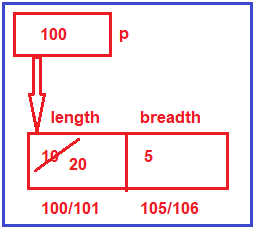In the next article, I am going to discuss Command Line Arguments in C Language with Examples. Here, in this article, I try to explain How to pass Structure as a Parameter to a function in C Language and I hope you enjoy this How to pass Structure as a Parameter to a function in the C Language article.